## A couple walking in the park strolls 1.5 km in 60 min. What is the couple’s average speed in m/h?

Question

A couple walking in the park strolls 1.5 km in 60 min. What is the couple’s average speed in m/h?

in progress 0
3 months 2021-07-31T17:37:25+00:00 2 Answers 5 views 0

0.932 m/h

Explanation:

Parameters given:

Distance walked by couple, D = 1.5 km

Time spent in walking, t = 60 min

To solve this we simply apply the formula for average peed:

Average Speed = total distance / total time taken

The question asks specifically to put the answer in miles per hour (m/h), hence, we need to convert the distance to miles and the time to hours:

Distance in miles:

1 km = 0.622 miles

1.5 km = 1.5 * 0.622 = 0.932 miles

Time in hours = 60 / 60 = 1 hour

Hence, the speed is:

Speed = 0.932 / 1 = 0.932 m/h

The average speed of the couple is 0.932 m/h

1,500m/h

Explanation:

the information that we have is:

distance traveled: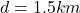to convert this quantity to meters we remember that 1km = 1,000m

so the distance in meters is: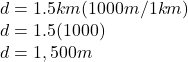and we also know the time

time: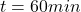, and since 60min = 1hour, the time in hours is: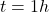now we use the formula for the average speed: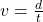and we sustitute the known values (since we want the answer to be in m/h we substitute the distance in meters (m) and the time in hours(h)):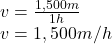the speed of the couple in m/h is: 1,500m/h